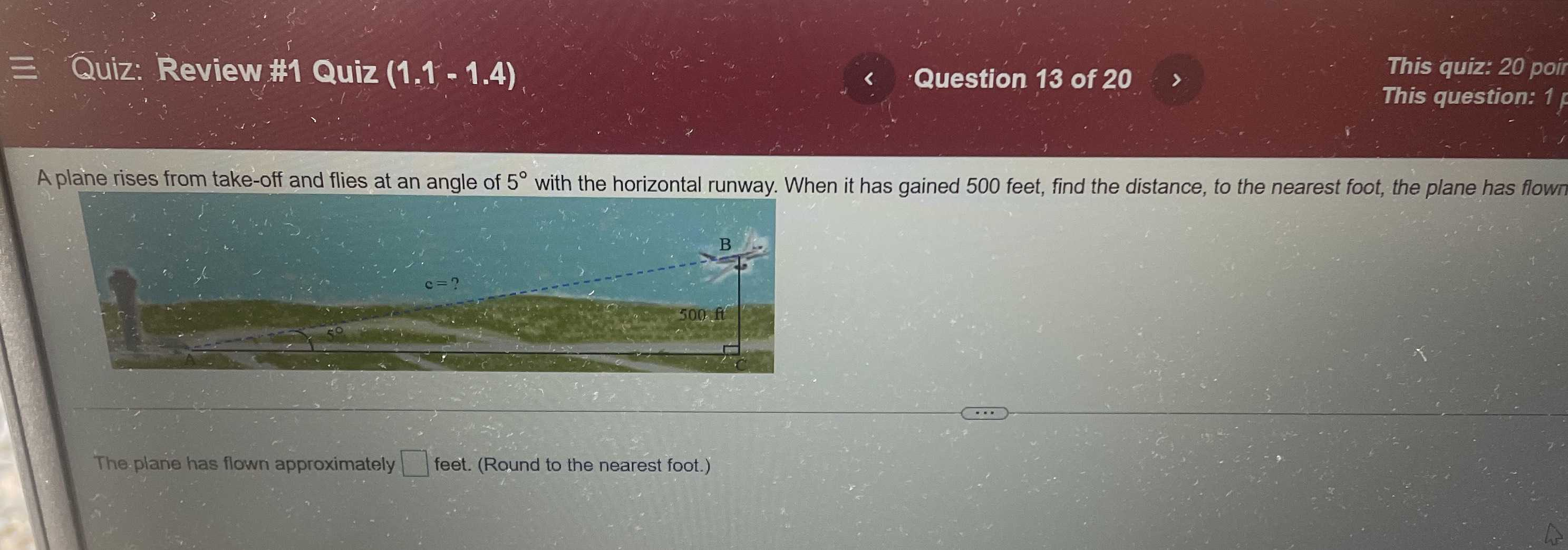### Still have math questions?

Trigonometry
QuestionA plane rises from take-off and flies at an angle of $$5 ^ { \circ }$$ with the horizontal runway. When it has gained $$500$$ feet, find the distance, to the nearest foot, the plane has flown

The.plane has flown approximately $$\square$$ feet. (Round to the nearest foot.)